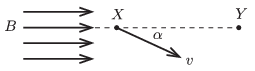Mathematical and Physical Journal
for High Schools
Issued by the MATFUND Foundation
 Already signed up? New to KöMaL?

#Problem P. 4744. (May 2015)

P. 4744. There are two points ($\displaystyle X$ and $\displaystyle Y$) on the same $\displaystyle B$-line at a distance of 10 cm in a uniform magnetic field of magnetic induction $\displaystyle B=0.02$ tesla. An electron, which was accelerated through a potential difference of 800 V, passes point $\displaystyle X$, such that its velocity encloses an angle of $\displaystyle \alpha$ with the induction line. What may the value of $\displaystyle \alpha$ be if the electron passes point $\displaystyle Y$ as well?(5 pont)

Deadline expired on June 10, 2015.

### Statistics:

 39 students sent a solution. 5 points: Asztalos Bogdán, Balogh Menyhért, Bartók Imre, Bekes Nándor, Berta Dénes, Blum Balázs, Bugár 123 Dávid, Büki Máté, Csathó Botond, Csire Roland, Csorba Benjámin, Fehér Balázs, Fekete Panna, Forrai Botond, Fülöp Erik, Holczer András, Iván Balázs, Jakus Balázs István, Kasza Bence, Körtefái Dóra, Marosvári Kristóf, Molnár 157 Marcell, Németh Flóra Boróka, Olosz Balázs, Öreg Botond, Radnai Bálint, Sal Kristóf, Szentivánszki Soma , Tibay Álmos, Trócsányi Péter. 4 points: Varga-Umbrich Eszter. 3 points: 4 students. 2 points: 1 student. 1 point: 2 students. Unfair, not evaluated: 1 solutions.

Problems in Physics of KöMaL, May 2015